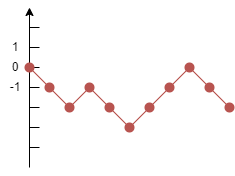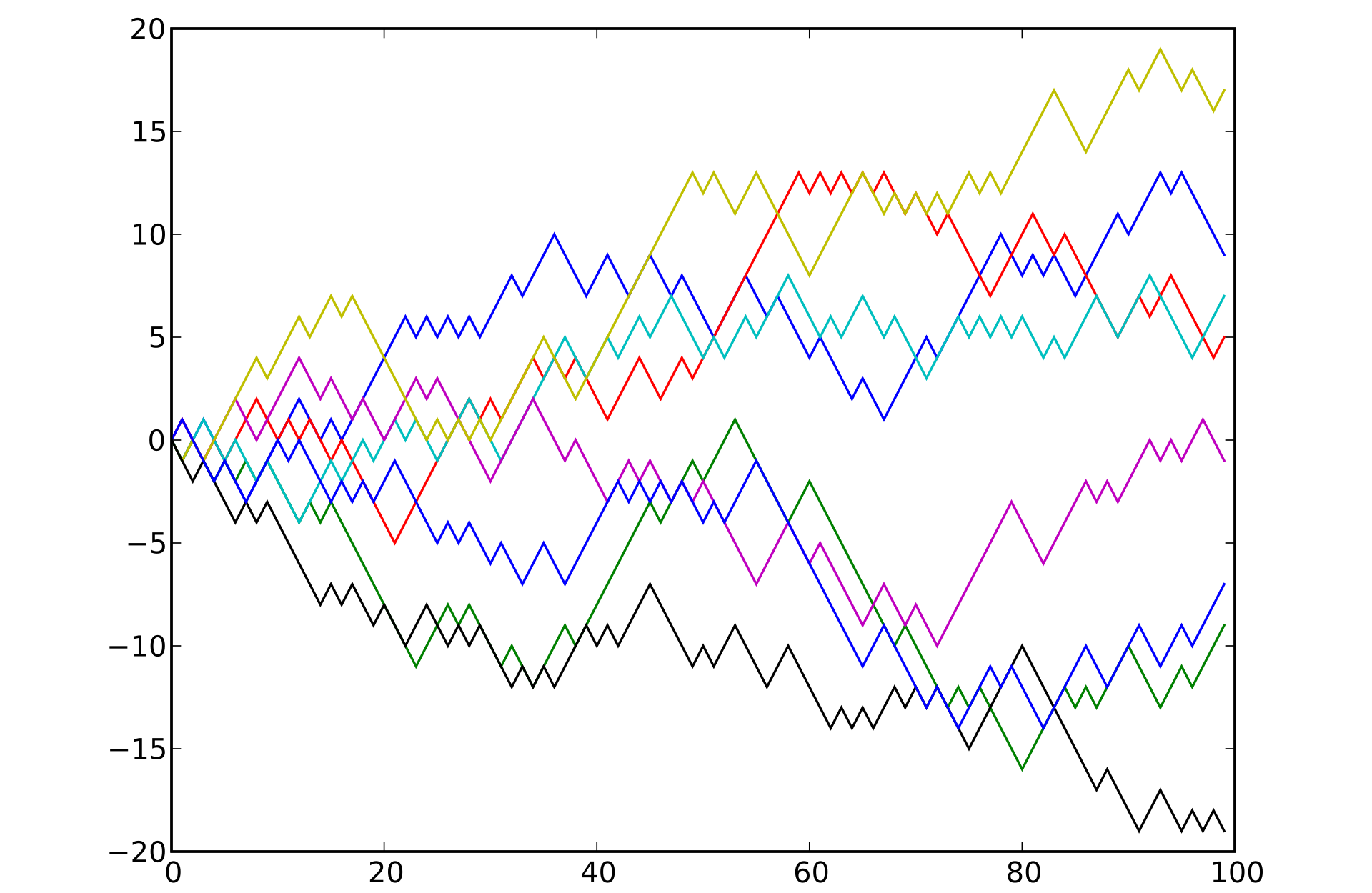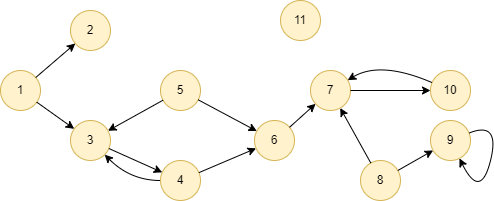# Change Nickname

Current Nickname:

Certifications

Certificate Issued at Valid until Serial No. File
Serial No. Valid until

Not having one? Apply now! >>>

Invoice

ProductName CreateTime ID Price File
ProductName CreateTime ID Price File

# Random Walk

## Overview

Random Walk, as the name suggests, refers to starting from a certain node (any or all) in the graph, traversing one of the adjacent edges randomly to reach another node, and repeating this process until all accessible nodes are reached or the limit of the defined depth or time for the traversal is met. The concept of 'random walk' was formally proposed by the British mathematician and biostatistician Karl Pearson in 1905:

## Basic Concept

### Random Walk

Random walk (RW) is a mathematical model. Each random walk produces a path, each path in the repeated random walks is formed randomly. It is used to represent irregular forms of change, like a random process record formed by a person's drunken steps.

An elementary random walk is performed on the 1-dimensional space: A node starts from the origin of the number line and moves up or down one unit at a time with equal probability, the path of a 10-step random walk is as follows:Perfrom this random walk multiple times, each time with 100 steps, the paths are illustarted below:### Random Walk in Graph

In the graph, a random walk refers to the process of forming a path by starting from a node and continuously passing through neighbor nodes, the process is regulated by the walk depth (i.e. the number of nodes to be visited) and some filtering conditions (generally, one or multiple properties of edge). Assuming there are 8 nodes in the graph, after performing 300 30-step random walks, 2,400 paths will be produced, and each path contains 30 nodes the maximum.

Ultipa's Random Walk algorithm performs the classic random walk: by default, each edge has the same weight (equals to 1) so that the probability being traversed is the same; when specifying edge weight, the probability of passing through these edges is proportional to the edge weight. In fact, there are many forms of random walks, such as Node2Vec walks, Struc2Vec walks, and GraphSAGE sampling.

## Special Case

### Lonely Node, Disconnected Graph

Lonely node has no adjacent node, hence it cannot walk to other nodes; lonely node can only walk along its self-loop edge if there is one. The walk paths of non-lonely nodes will not have any lonely node.

Node only walks within its own connected component.

### Self-loop Edge

Node may walk along its self-loop edge.

### Directed Edge

The direction of edge is ignored when performing random walks.

## Results and Statistics

Perform random walk in the whole graph below for 2 times with a depth of 6, each edge weight is 1:Algorithm results: There are 11 nodes in the graph, thus 11*2 = 22 node arrays are contained in the returned `walks`

walks
[5, 6, 4, 3, 5, 6]
[6, 5, 3, 5, 6, 5]

[10, 7, 8, 7, 10, 7]
[8, 9, 9, 9, 9, 9]
[7, 10, 7, 10, 7, 10]
[2, 1, 2, 1, 2, 1]
[9, 9, 9, 8, 7, 6]
[1, 3, 4, 6, 4, 3]
[3, 5, 3, 1, 3, 1]
[4, 3, 4, 3, 4, 3]
[5, 3, 4, 6, 5, 6]
[6, 5, 6, 5, 6, 7]

[10, 7, 8, 7, 10, 7]
[8, 9, 8, 7, 10, 7]
[7, 10, 7, 6, 5, 6]
[2, 1, 3, 4, 6, 4]
[9, 8, 9, 9, 9, 9]
[1, 3, 4, 3, 5, 6]
[3, 4, 3, 4, 6, 7]
[4, 3, 1, 3, 1, 2]

Algorithm statistics: N/A

## Command and Configuration

• Command：`algo(random_walk)`
• Configurations for the parameter `params()`:
Name Type
Default
Specification
Description
ids / uuids []`_id` / []`_uuid` / / IDs or UUIDs of nodes to start the walk; all nodes to be selected if not set
walk_length int 1 >=1 Depth of each walk, i.e. the number of nodes to visit
walk_num int 1 >=1 Number of walks
edge_schema_property []`@<schema>?.<property>` / Numeric edge property, LTE needed Edge weight property/properties, schema can be either carried or not; nodes only walk along edges with the specified properties and the probability of passing through these edges is proportional to the edge weight; if edge has multiple specified properties, the edge weight is the sum of these property values; the weight of all edges is 1 if not set
limit int -1 >=-1 Number of results to return; return all results if sets to -1 or not set

Example: Select nodes with UUID = 1,2,...,10 to perform random walk for 2 times with a depth of 6

``````algo(random_walk).params({
uuids: [1,2,3,4,5,6,7,8,9,10],
walk_length: 6,
walk_num: 2
}) as walks
return walks
``````

## Algorithm Execution

#### 1. File Writeback

Configuration
Data in Each Row
Description
filename `_id`,`_id`,... IDs of nodes that walked through

Example: Select nodes with UUID = 1,2,...,10 to perform random walk for 2 times with a depth of 6, write the algorithm results back to file named path

``````algo(random_walk).params({
uuids: [1,2,3,4,5,6,7,8,9,10],
walk_length: 6,
walk_num: 2
}).write({
file:{
filename: "path"
}})
``````

#### 2. Property Writeback

Not supported by this algorithm.

#### 3. Statistics Writeback

This algorithm has no statistics.

### Direct Return

Alias Ordinal Type
Description
Column Name
0 []perWalk Array of UUIDs of nodes that walked through each time `[_uuid, _uuid, ...]`

Example: Perform random walk in the whole graph for 3 times with a depth of 5, define algorithm results as alias named path, and return the results

``````algo(random_walk).params({
walk_length: 5,
walk_num: 3
}).write({
file:{
filename: "path"
}})
``````

### Streaming Return

Alias Ordinal Type
Description
Column Name
0 []perWalk Array of UUIDs of nodes that walked through each time `[_uuid, _uuid, ...]`

Example: Perform random walk in the whole graph for 3 times with a depth of 5, and specify edge weight locates on property @follow.level, return the results that walked less than 5 steps

``````algo(random_walk).params({
walk_length: 5,
walk_num: 3,
edge_schema_property: @follow.level
}).stream() as walk
where size(walk) < 5
return walk
``````

### Real-time Statistics

This algorithm has no statistics.